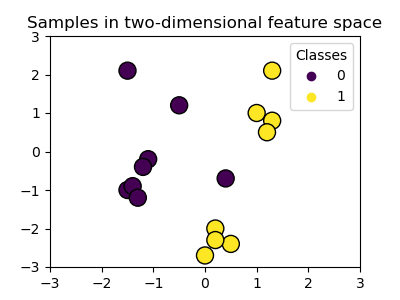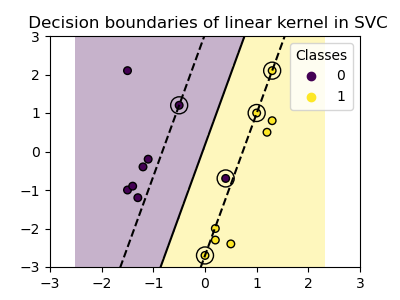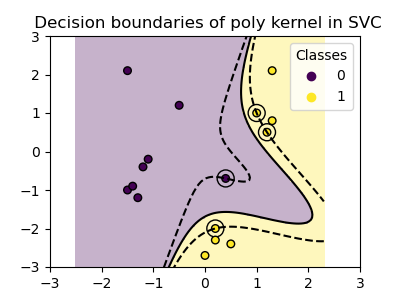# SVM-Kernels¶

Three different types of SVM-Kernels are displayed below. The polynomial and RBF are especially useful when the data-points are not linearly separable.

•••Out:

/home/circleci/project/examples/svm/plot_svm_kernels.py:78: MatplotlibDeprecationWarning: shading='flat' when X and Y have the same dimensions as C is deprecated since 3.3.  Either specify the corners of the quadrilaterals with X and Y, or pass shading='auto', 'nearest' or 'gouraud', or set rcParams['pcolor.shading'].  This will become an error two minor releases later.
plt.pcolormesh(XX, YY, Z > 0, cmap=plt.cm.Paired)
/home/circleci/project/examples/svm/plot_svm_kernels.py:78: MatplotlibDeprecationWarning: shading='flat' when X and Y have the same dimensions as C is deprecated since 3.3.  Either specify the corners of the quadrilaterals with X and Y, or pass shading='auto', 'nearest' or 'gouraud', or set rcParams['pcolor.shading'].  This will become an error two minor releases later.
plt.pcolormesh(XX, YY, Z > 0, cmap=plt.cm.Paired)
/home/circleci/project/examples/svm/plot_svm_kernels.py:78: MatplotlibDeprecationWarning: shading='flat' when X and Y have the same dimensions as C is deprecated since 3.3.  Either specify the corners of the quadrilaterals with X and Y, or pass shading='auto', 'nearest' or 'gouraud', or set rcParams['pcolor.shading'].  This will become an error two minor releases later.
plt.pcolormesh(XX, YY, Z > 0, cmap=plt.cm.Paired)


# Code source: Gaël Varoquaux

import numpy as np
import matplotlib.pyplot as plt
from sklearn import svm

# Our dataset and targets
X = np.c_[
(0.4, -0.7),
(-1.5, -1),
(-1.4, -0.9),
(-1.3, -1.2),
(-1.1, -0.2),
(-1.2, -0.4),
(-0.5, 1.2),
(-1.5, 2.1),
(1, 1),
# --
(1.3, 0.8),
(1.2, 0.5),
(0.2, -2),
(0.5, -2.4),
(0.2, -2.3),
(0, -2.7),
(1.3, 2.1),
].T
Y =  * 8 +  * 8

# figure number
fignum = 1

# fit the model
for kernel in ("linear", "poly", "rbf"):
clf = svm.SVC(kernel=kernel, gamma=2)
clf.fit(X, Y)

# plot the line, the points, and the nearest vectors to the plane
plt.figure(fignum, figsize=(4, 3))
plt.clf()

plt.scatter(
clf.support_vectors_[:, 0],
clf.support_vectors_[:, 1],
s=80,
facecolors="none",
zorder=10,
edgecolors="k",
)
plt.scatter(X[:, 0], X[:, 1], c=Y, zorder=10, cmap=plt.cm.Paired, edgecolors="k")

plt.axis("tight")
x_min = -3
x_max = 3
y_min = -3
y_max = 3

XX, YY = np.mgrid[x_min:x_max:200j, y_min:y_max:200j]
Z = clf.decision_function(np.c_[XX.ravel(), YY.ravel()])

# Put the result into a color plot
Z = Z.reshape(XX.shape)
plt.figure(fignum, figsize=(4, 3))
plt.pcolormesh(XX, YY, Z > 0, cmap=plt.cm.Paired)
plt.contour(
XX,
YY,
Z,
colors=["k", "k", "k"],
linestyles=["--", "-", "--"],
levels=[-0.5, 0, 0.5],
)

plt.xlim(x_min, x_max)
plt.ylim(y_min, y_max)

plt.xticks(())
plt.yticks(())
fignum = fignum + 1
plt.show()


Total running time of the script: ( 0 minutes 0.349 seconds)

Gallery generated by Sphinx-Gallery